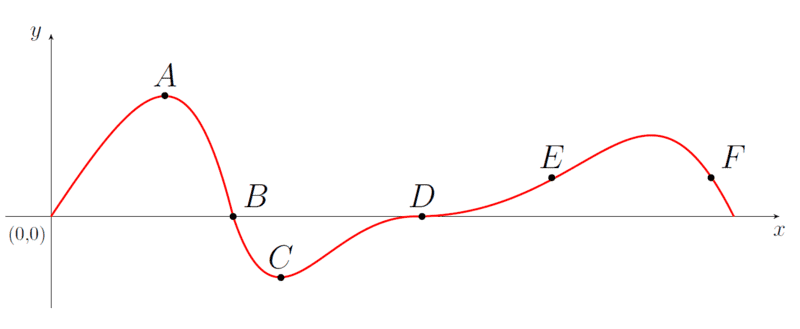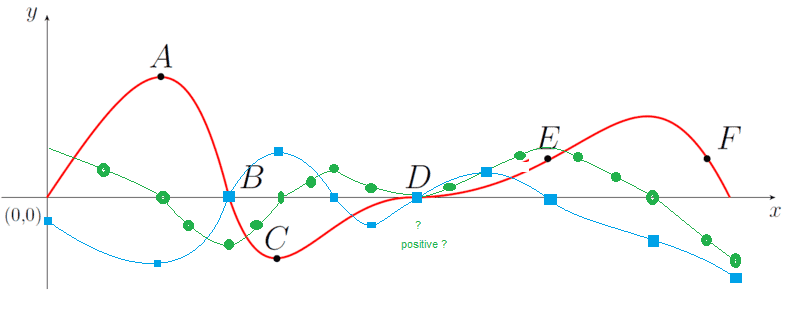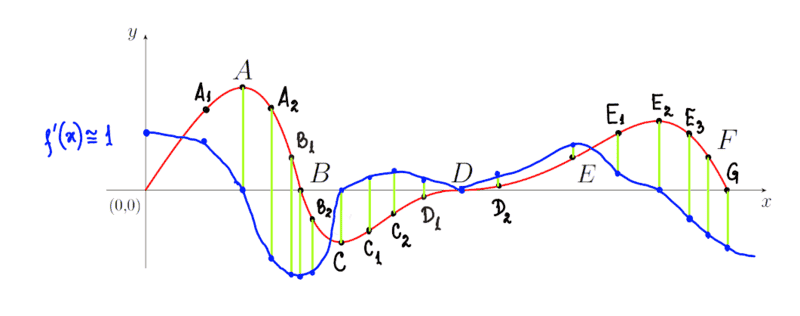# The first and second derivatives at various points on a drawn graph

brotherbobby
Homework Statement:
The figure below is the graph of a function ##y = f(x)##. Answer the following questions : ##\\[10pt]##
1. Locate the points at which the ##\text{first derivative}## of ##y## with respect to ##x## is ##\text{non-zero}##.##\\[5pt]##
2. Locate the points at which the ##\text{second derivative}## of ##y## with respect to ##x## is ##\text{non-zero}##. ##\\[10pt]##
Relevant Equations:
1. Given a function ##f(x)##, the first derivative ##f'(x)## is 0 at a point of local extremum (maxima, minima or point of inflexion, also called saddle points). ##\\[5pt]##
2. Given a function ##f(x)##, the second derivative ##f''(x)## is negative or positive at a point of maxima or minima, respectively. At a saddle point, ##f''(x)## is zero also. ##\\[10pt]##Problem statement :
The function ##y = f(x)## is given above.

Question 1 : Locate the points at which the ##\text{first derivative}## of ##y## with respect to ##x## is ##\text{non-zero}##.##\\[5pt]##

At points of extrema, like A, C and D, the derivative is zero. Hence the derivative is non zero at the remaining points : ##\boxed{B, E, F}##.

Question 2 : Locate the points at which the ##\text{second derivative}## of ##y## with respect to ##x## is ##\text{non-zero}##. ##\\[10pt]##

Now this is the more difficult bit, because no graph of the derivative ##f'(x)## is given. However, we know that at ##A## (a maxima), derivative is negative, hence non-zero##\huge{\checkmark}##. At ##C## (a minima), derivative is positive, hence non-zero##\huge{\checkmark}##. At saddle point ##D##, second derivative (and first derivative) is zero. It gets a bit tricky at points ##B, E, F##. At ##B##, ##f'(x)## is negative and (looks to) remain the same before and after. So if we assume ##f'(B)## to be locally constant, ##f''(B)## must be zero. ##\huge{\times}## The same can be said for points ##E,F##; at all these points, the function ##f(x)## is either monotically increasing or decreasing implying that the derivative is constant and that the second derivative is zero. ##\huge{\times}##

So my answer is, at points ##\boxed{A,C}## are the second derivatives of the function non-zero.

Are my answers correct? A hint would be welcome.

Staff Emeritus
Homework Helper
Gold Member
For a local extremum, the second derivative will tell you whether or not it is a max or min (or if it is zero - it could be either or a saddle point). This does not mean that it is zero away from all minima. The second derivative is zero if the graph does not curve at the given point.

Gold Member
Points where the second derivative is zero can be points where the concavity of the function change ...
Ssnow

•Delta2
Homework Helper
Gold Member
I agree with your answer to part 1 but disagree with your answer to part 2. Your descriptions of the first and second derivatives are not very good. There is more to say than how they behave at extreme points. I would say that the first derivative is the slope and the second derivative is the curvature. Try using those descriptions to answer the questions.

brotherbobby
For a local extremum, the second derivative will tell you whether or not it is a max or min
@Orodruin - Yes, once I have been able to ascertain that it is an extremum, by virtue of the vanishing of the first derivative, the second derivative can tell me if it is a maximum or minimum. But you go on to add :
(or if it is zero - it could be either or a saddle point)
If it is zero? Yes it can be a saddle point, but could it be either - a maximum or minimum and be zero? Well I suppose if we have a graph like ##y = 3##, the second derivative is zero all over and yet, every point is a minimum and a maximum. Did you mean something else?

This does not mean that it is zero away from all minima.
Yes, I understand. Or maxima. Just because the point is not an extremum doesn't mean the second derivative has to be zero at that point.

The second derivative is zero if the graph does not curve at the given point.
Yes again. If the graph does not curve, the first derivative is a constant, meaning its derivative (the 2nd) must be zero.

Please let me know, when you can, whether my understanding of your statements were correct. They are very important statements, not always spelt out clearly in books.

brotherbobby
Points where the second derivative is zero can be points where the concavity of the function change ...
Yes I have read that, but didn't understand it. Can you elaborate? I am aware that concavity or convexity is about whether a graph "curves up" or curves down". For example, the function ##y = x^2## is concave at ##x=0##. Likewise the graph ##y=-x^2## is convex at ##x=0##.

brotherbobby
I agree with your answer to part 1 but disagree with your answer to part 2. Your descriptions of the first and second derivatives are not very good. There is more to say than how they behave at extreme points. I would say that the first derivative is the slope and the second derivative is the curvature. Try using those descriptions to answer the questions.
The second derivative is a bit hard to visualise because it's a slope of a slope. But yes, it tells us as to how a function curves. I can understand how given a function ##y = x^2##, the second derivative is positive everywhere. That is because even though the first derivative (##2x##) is negative below zero and positive above zero, it is everywhere increasing. Also this graph is concave, so perhaps a positive second derivative has to do with concavitity and vice versa.Yes, my first answer about only the derivative is correct, as I have checked from the website later. Let me put the graph here again. For the second question, the book says that point ##F## has second derivative non-zero, along with points ##A,C## (which I got). Far as I can see, at ##F##, the slope is constant around it, much like at point ##B##, hence the second derivative has to be zero. Or, taking your definition, the graph does not curve at ##F##.

Please let me know if am wrong when you have the time.

Homework Helper
Gold Member
View attachment 283378 Or, taking your definition, the graph does not curve at ##F##.

Please let me know if am wrong when you have the time.
I think that if you look closely, you would see that there is some curvature at F. It is not exactly straight there.

•pbuk
Staff Emeritus
Homework Helper
Gold Member
If it is zero? Yes it can be a saddle point, but could it be either - a maximum or minimum and be zero? Well I suppose if we have a graph like y=3, the second derivative is zero all over and yet, every point is a minimum and a maximum. Did you mean something else?
You will have to continue to check the higher derivatives to establish the type of extremum. For example, ##x^3## has a saddle point at ##x=0## because the first non-vanishing derivative is odd whereas ##x^4## has a minimum because the first non-vanishing derivative is even and positive.
Yes again. If the graph does not curve, the first derivative is a constant, meaning its derivative (the 2nd) must be zero.
No, as has been pointed out, this is incorrect. ”Not curve” here means to change concavity.

Homework Helper
Gold Member
Yes I have read that, but didn't understand it. Can you elaborate? I am aware that concavity or convexity is about whether a graph "curves up" or curves down". For example, the function ##y = x^2## is concave at ##x=0##. Likewise the graph ##y=-x^2## is convex at ##x=0##.

No you have this the wrong way round, and furthermore ##y = x^2## is (strictly) convex everywhere, not just at ##x=0## (and ##y=-x^2## is strictly concave everywhere).

If you have trouble remembering which way round it is, add the word 'downwards': ##y = x^2## is convex downwards and ##y = -x^2## is concave downwards.

brotherbobby
I think that if you look closely, you would see that there is some curvature at F. It is not exactly straight there.
Yes I was thinking this must be the solution to the problem. I am afraid a problem of this sort - "deciding on derivatives from the picture of a graph" - is not unambiguous. It is difficult to ascertain how closely should one look. Or whether the graph is totally straight or slightly not.

•FactChecker
brotherbobby
You will have to continue to check the higher derivatives to establish the type of extremum. For example, ##x^3## has a saddle point at ##x=0## because the first non-vanishing derivative is odd whereas ##x^4## has a minimum because the first non-vanishing derivative is even and positive.

No, as has been pointed out, this is incorrect. ”Not curve” here means to change concavity.
So you mean that a graph "does not curve" if it "changes concavity"?

Homework Helper
Gold Member
Yes I was thinking this must be the solution to the problem. I am afraid a problem of this sort - "deciding on derivatives from the picture of a graph" - is not unambiguous. It is difficult to ascertain how closely should one look. Or whether the graph is totally straight or slightly not.
I agree. But I think that if you look closely at B, D, and E, you would say that the curve is one way on one side and the other way on the other side. So those points are close to a zero point of the second derivative. On the other hand, the curvature is the same on both sides of A, C, and F, so you would not guess that those points are zeros of the second derivative.

MidgetDwarf
Make a graph of the first derivative. Do this first.

Homework Helper
Gold Member
I'm no mathematician, but we used to do curve sketching. You just mark some obvious points then join them up in approx the right sort of way.
Here green is 1st deriv, then blue is 2nd deriv obtained as 1st deriv of green sketch.
This is a quick first attempt in Paint, so not very accurate, but the zeros are preetty obvious.

At D, I can't see any reason why green has to be zero, but it's a minimum, so blue is zero.

Similarly at B, I can't see any reason why the inflexion between A and C of the red line has to be at B (the zero of red), so the blue zero (and green minimum) probably should be slightly away from B. (This is not shown, because I didn't realize this at first.)

The zero and max at E are not uncertain, because the only reason to put E there is to mark the max slope.Last edited:
•Delta2 and FactChecker
brotherbobby
I'm no mathematician, but we used to do curve sketching. You just mark some obvious points then join them up in approx the right sort of way.
Here green is 1st deriv, then blue is 2nd deriv obtained as 1st deriv of green sketch.
This is a quick first attempt in Paint, so not very accurate, but the zeros are preetty obvious.

At D, I can't see any reason why green has to be zero, but it's a minimum, so blue is zero.

Similarly at B, I can't see any reason why the inflexion between A and C of the red line has to be at B (the zero of red), so the blue zero (and green minimum) probably should be slightly away from B. (This is not shown, because I didn't realize this at first.)

The zero and max at E are not uncertain, because the only reason to put E there is to mark the max slope.
https://www.physicsforums.com/attachments/283439
I do not want to see both your first (green) and second derivatives (blue) in the graph just yet but would rather try and sketch them myself. This was suggested to me in post# 14 above by @MidgetDwarf. I am excited about this because I have never done it myself before!

Homework Helper
Gold Member
Apologies. I hadn't remembered this was a HW Q.
I've deleted it for now.
I see some people can hide things with "Spoiler Alert" but I don't know how to do that.

Staff Emeritus
Homework Helper
Gold Member
I see some people can hide things with "Spoiler Alert" but I don't know how to do that.

In the rows of symbols above the editing field (click the three dots if all do not appear), click the eye with the diagonal line across.•SammyS
Homework Helper
Gold Member
@Orodruin Thanks very much. Now I can also post in What I learned Today.

MidgetDwarf
I do not want to see both your first (green) and second derivatives (blue) in the graph just yet but would rather try and sketch them myself. This was suggested to me in post# 14 above by @MidgetDwarf. I am excited about this because I have never done it myself before!
The idea is to draw a few tangents along the given curve. We know that at max and mins, the derivative is equal to zero. Now, the idea is to see how the tangents are changing near the max/min (if any).

I'll hold off on another hint. Let me know, I can draw a quick portion if you would like.

brotherbobby
Make a graph of the first derivative. Do this first.I have never done this but willing to try. This has been done in post#15 above by @Merlin3189 but I would only refer to it if I struggle.
Problem : Let me start by putting the image first, to the right. (I hope it's big enough to see).
Question : I would answer the original questions later (see my post# 1 above). For now the question is : ##\text{Given the graph of the function}\; f(x)\;, \text{draw the graph of its derivative}\;f'(x)\; \text{over the same domain}##.

Attempt : I would like to take this in intervals, if you don't mind. Of course it shows I am tentative. I find the derivative(s) by deciding on the "amount" of the slope the tangent has at the point in question. For local maxima and minima I am helped by the fact that the derivative is vanishing. For near straight paths, the derivative remains almost constant.Please let me know how well have I done, when you can. Thanks for your time and patience.

brotherbobby
the idea is to see how the tangents are changing near the max/min (if any).

I'll hold off on another hint.
@MidgetDwarf - If the moment is right given by drawing of ##f'(x)## above for the given function, I would like to know what your second hint was.